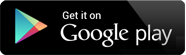## Video Lectures

Chapter 1 : Introduction to Discrete Mathematics
Topic : Variables
Content : Mathematical Statements 19 MB ,15:0 MIN , THEORY

Chapter 1 : Introduction to Discrete Mathematics
Topic : The Language of Sets
Content : The Language of Relations and Functions 51 MB ,39:30 MIN , THEORY

Chapter 2 : Set theory
Topic : Definitions and Element method of proof
Content : Definitions of Equal sets, null sets, singleton sets etc 41 MB ,32:58 MIN , THEORY

Chapter 2 : Set theory
Topic : Properties of Sets, Disproof, Algebraic proofs
Content : Operations on sets, Laws on sets 80 MB ,1:0:29 MIN , THEORY

Chapter 2 : Set theory
Topic : Boolean Algebras
Content : Properties with proof 82 MB ,1:2:26 MIN , THEORY

Chapter 2 : Set theory
Topic : Russell's Paradox, The Halting Problem
Content : Examples Of Boolean Algebra 62 MB ,46:36 MIN , THEORY

Chapter 2 : Set theory
Topic : Problems related to set theory
Content : Problems on cartesian product, algebraic properties 53 MB ,38:58 MIN , MATHS SOLUTION

Chapter 3 : The logic of compound statements
Topic : Logical form and Logical Equivalence, Conditional Statements
Content : Bi-Conditional Statement 87 MB ,1:5:23 MIN , THEORY

Chapter 3 : The logic of compound statements
Topic : Problems on Logical form and logical Equivalence
Content : Drawing Truth table, Finding Negations 52 MB ,39:52 MIN , THEORY

Chapter 3 : The logic of compound statements
Topic : Valid and Invalid arguments
Content : Some types of logical arguments, example of valid and invalid arguments 42 MB ,29:7 MIN , THEORY

Chapter 1 : Quantified Statements Structure
Topic : Predicates and Quantified Statements, Statements with multiple Quantifiers, Arguments with Quantified Statements
Content : Negation of Quantified Statements, Different variants of universal conditional statements 95 MB ,1:6:5 MIN , THEORY

Chapter 2 : Elementary Number Theory & Methods of Proof
Topic : Introduction to direct proof, Rational Numbers, Divisibility, Division into cases
Content : Proving Existential and Universal Statements, Disproving Universal Statements by Counterexample 131 MB ,1:32:4 MIN , THEORY

Chapter 2 : Elementary Number Theory & Methods of Proof
Topic : Quotient-Remainder Theorem, Floor and ceiling Functions, Indirect Arguments: Contradiction and Contraposition, Two Classical Theorem, Application in Algorithms
Content : Parity of integer, Euclidean Algorithm, greatest common divisor 88 MB ,1:1:14 MIN , THEORY

Chapter 1 : Sequences, Mathematical Induction & Recursion
Topic : Sequences, Mathematical Induction, Strong Mathematical Induction
Content : Summation, Product and factorial notation. closed forms 59 MB ,42:9 MIN , THEORY

Chapter 1 : Sequences, Mathematical Induction & Recursion
Topic : The Well ordering principle for the integers, Correctness of Algorithm, Defining Sequences recursively, Second order linear Homogeneous Recurrence Relations with constant Coefficient, General Recursive Definitions and Structural Induction
Content : Quotient-Remainder theorem 72 MB ,48:47 MIN , THEORY

Chapter 2 : Functions
Topic : Functions defined on General Sets, One-to-One and Onto, Inverse Functions, Composition of Functions
Content : Range, Domain, Co-domain, Image, Bijective Functions 73 MB ,53:59 MIN , THEORY

Chapter 2 : Functions
Topic : Cardinality with Applications to Computability
Content : Properties of Cardinality, Countable Sets and Uncountable Sets 27 MB ,19:21 MIN , THEORY

Chapter 1 : Relations
Topic : Relations on Sets
Content : Inverse of a Relation, Composition of Relation, Matrix Representation, Diagraph 76 MB ,53:56 MIN , THEORY

Chapter 1 : Relations
Topic : Reflexivity, Symmetry and Transitivity, Equivalence Relations, Partial order Relations
Content : Empty Relation, Anti-Symmetric Relation, POSET, Hasse Diagrams 82 MB ,58:34 MIN , THEORY

Chapter 2 : Graphs and Trees
Topic : Definitions and basic properties, Matrix representation of Graphs, Isomorphism of graphs, Trails, Paths and Circuits
Content : Some graphs, Hand shaking lemma, Connectedness, Euler circuit, Hamiltonian Circuit, Planar Graph 87 MB ,59:8 MIN , THEORY

Chapter 2 : Graphs and Trees
Topic : Trees, Rooted Trees, Spanning trees and Shortest paths
Content : Properties of Tree graph, Prim's Algorithm, Kruskal's Algorithm 48 MB ,32:37 MIN , THEORY

Chapter 1 : Counting and Probability
Topic : Introduction, Possibility Trees and Multiplication rule, Counting elements of disjoint sets: The addition rule, The pigeonhole Principle, Counting subsets of a set: r-combination with repetition allowed
Content : Subtraction rule, Generalized Pigeonhole principle 44 MB ,29:48 MIN , THEORY

Chapter 1 : Counting and Probability
Topic : Probability Axioms and Expected value, Conditional Probability, Baye's Formula and Independent events
Content : Random Experiments, Types of events 44 MB ,31:14 MIN , THEORY

## The Shikshak App

The Shikshak App is an honest attempt to provide quality education.